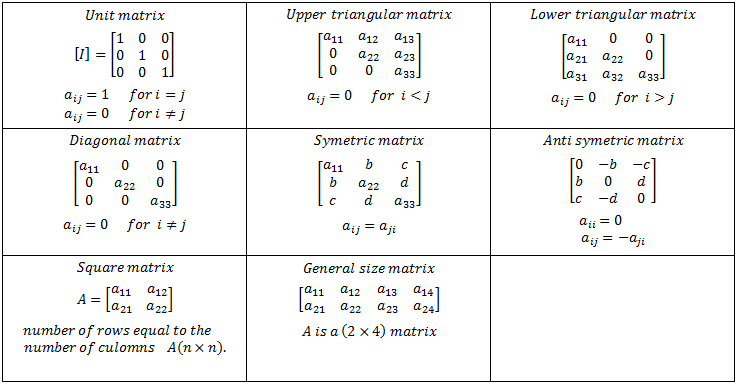# Types of Matrices

### Row Matrix

A matrix having only one row is called a row matrix or a row vector.

A = [aij]1×n

### Column Matrix

A matrix having only one column is called a column matrix or a column vector.

A = [aij]m×1

Any matrix of order 1×1 can be treated as either a row matrix or a column matrix.

### Square Matrix

A square matrix is a matrix in which the number of rows and the number of columns are equal. A matrix of order n×n is also known as a square matrix of order n.

A = [aij]n×n

The elements a11, a22, a33 … ann are called principal diagonal or leading diagonal or main diagonal elements.

The number of elements in a square matrix of order n is n2.

### Diagonal Matrix

A square matrix A = [aij]n×n is said to be a diagonal matrix if aij = 0 when i ≠ j.

In a diagonal matrix, all the entries except the entries along the main diagonal are zero.

### Triangular Matrix

A square matrix in which all the entries above the main diagonal are zero is called a lower triangular matrix. If all the entries below the main diagonal are zero, it is called an upper triangular matrix.

### Scalar Matrix

A square matrix A = [aij]n×n is said to be scalar matrix if aij = a if i = j and aij = 0 if i ≠ j.

A scalar matrix is a diagonal matrix in which all the entries along the main diagonal are equal.

### Identity Matrix or Unit Matrix

An identity matrix or a unit matrix is a scalar matrix in which entries along the main diagonal are equal to 1. the identity matrix of order n is represented as In.

### Zero Matrix or Null Matrix or Void Matrix

A matrix is said to be a zero matrix or null matrix if all the entries are zero, and is denoted by O.